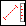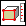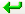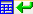> > To measure the perimeter of a face

To measure the perimeter of a face
Measure the perimeter of a face as follows:
1. Click Analysis and then, in the Measure group, click the arrow next toDistance.
2. ClickPerimeter. The Measure Output and Distance dialog boxes open.
3. Click the face to be measured.
4. The application displays the perimeter of the face in the Distance Output field.
5. Click the green arrow buttonto send the measurement to a command.
6. Click the green arrow and calculator buttonto send the measurement to the calculator.
If you attempt to measure the perimeter of a cylinder face, the resulting measurement will be the sum of the perimeters of both circular cylinder ends.